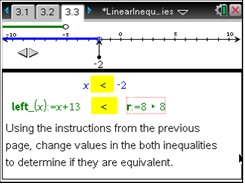# Activities

••• ##### Subject Area

• Math: Algebra I: Equations and Inequalities
• Math: Developmental Mathematics - College: Real Numbers and Linear Equations

• ##### Author9-12

75 Minutes

• ##### Device
•TI-Nspire™ CX/CX II
•TI-Nspire™ CX CAS/CX II CAS
• TI-Nspire™
• TI-Nspire™ CAS
• ##### Software

TI-Nspire™
TI-Nspire™ CAS

3.0

## Linear Inequalities

#### Activity Overview

Students first look at tables of values to see that inequalities are true for some values of the variable and not for others. They then graph simple inequalities, comparing the handheld output with graphs they create on paper. The last two problems have students solve one-step linear inequalities using addition, subtraction, multiplication, or division.

#### Key Steps

•In this problem, students explore a table of values for the inequality x ≥ 4. The table is set up to display the values of the variable, the left side of the inequality, and the right side of the inequality (Columns A, B, and C, respectively). Students are guided by a series of questions on their worksheet to discover that Column D, titled boolean, displays a 1 if the inequality is true for the given x-value or a 0 if the inequality is false.